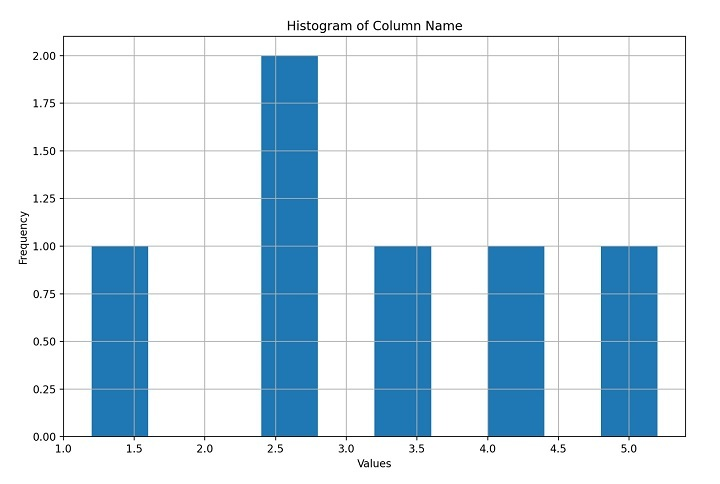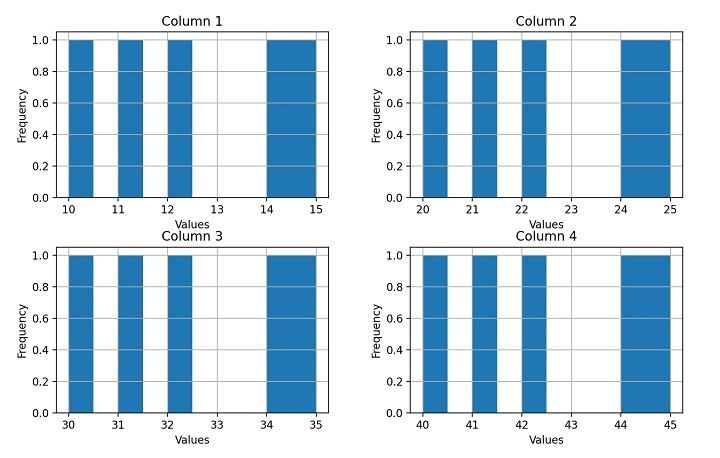# How to Create a Histogram from Pandas DataFrame?

A histogram is a graphical representation of the distribution of a dataset. It is a powerful tool for visualizing the shape, spread, and central tendency of a dataset. Histograms are commonly used in data analysis, statistics, and machine learning to identify patterns, anomalies, and trends in data.

Pandas is a popular data manipulation and analysis library in Python. It provides a variety of functions and tools to work with structured data, including reading, writing, filtering, cleaning, and transforming data. Pandas also integrates well with other data visualization libraries such as Matplotlib, Seaborn, and Plotly.

To create a histogram from a Pandas DataFrame, we first need to extract the data we want to plot. We can do this by selecting a column from the DataFrame using its name or index. Once we have the data, we can pass it to a histogram function from a visualization library to generate the plot.

There are several ways to create a histogram from a Pandas DataFrame using different visualization libraries. For example, we can use the "hist" method from Pandas, the "histogram" function from NumPy, or the "distplot" function from Seaborn. We can also customize the appearance of the histogram by changing the colour, bins, title, axis labels, and other properties.

## Syntax

We will be using the following syntax for creating a histogram from Pandas DataFrame.

DataFrame.hist(column=None, by=None, grid=True, xlabelsize=None, xrot=None, ylabelsize=None, yrot=None, ax=None, sharex=False, sharey=False, figsize=None, layout=None, bins=10, backend=None, legend=False, **kwargs)


## Explanation

Here is an explanation of the main parameters −

• column  The name or index of the column to plot. If None, all columns are plotted.

• by  The name or index of the column to group the data by. If provided, multiple histograms are created, one for each group.

• grid  Whether to show grid lines on the plot.

• xlabelsize, xrot, ylabelsize, yrot  Size and rotation of the x-axis and y-axis labels.

• ax  Matplotlib axis object to plot on. If None, a new axis is created.

• sharex, sharey  Whether to share the x-axis or y-axis among the subplots.

• figsize  Size of the figure in inches (width, height).

• layout  (rows, columns) of the subplot layout. If provided, the "by" parameter is ignored.

• bins  The number of bins to use for the histogram. This can be an integer or a sequence of bin edges.

• backend  The plotting backend to use, such as 'matplotlib' or 'plotly'.

• legend −  Whether to show the legend on the plot.

Now let's explore the examples where we will be creating these histograms.

## Single Column Histogram

A single column histogram in Python is a graphical representation of the frequency distribution of a dataset with only one column of data. Consider the code shown below.

import pandas as pd
import matplotlib.pyplot as plt

# Read the CSV file into a DataFrame

# Plot a histogram of a single column in the DataFrame
df.hist(column='column_name')

# Set the title and axis labels
plt.title('Histogram of Column Name')
plt.xlabel('Values')
plt.ylabel('Frequency')

# Display the histogram
plt.show()


## Explanation

• Import the necessary libraries, including pandas and matplotlib.pyplot.

• Read the CSV file into a Pandas DataFrame using the pd.read_csv() function.

• Use the df.hist() function to plot a histogram of a single column in the DataFrame.

• Set the title and axis labels using the plt.title(), plt.xlabel(), and plt.ylabel() functions.

• Display the histogram using the plt.show() function.

To run the above code, you need to install the pandas and matplotlib library, and for that, you can use following command −

pip3 install pandas matplotlib


## Output

Once pandas and matplotlib is installed successfully, you can execute the code and it will produce the following histogram −## Multiple Column Histogram

A multiple column histogram in Python is a graphical representation of the frequency distribution of a dataset with multiple columns of data. Consider the code shown below.

import pandas as pd
import matplotlib.pyplot as plt

# Read the CSV file into a DataFrame

# Plot histograms of all columns in the DataFrame
df.hist()

# Set the title and axis labels for each histogram
for ax in plt.gcf().axes:
ax.set_title(ax.get_title().replace('Histogram of ', ''))
ax.set_xlabel('Values')
ax.set_ylabel('Frequency')

# Display the histograms
plt.show()


## Explanation

This Python code reads a CSV file and plots histograms for all columns in the file using Pandas and Matplotlib. It then sets the titles and axis labels for each histogram before displaying them on the screen.

## Output

On execution, it will produce the following output −## Conclusion

In conclusion, creating a histogram from a Pandas DataFrame is a simple and effective way to visualize the distribution of data. With the help of the Pandas and Matplotlib libraries, you can quickly create a histogram for a single column or multiple columns of data in a DataFrame, customize the appearance of the histogram, and add axis labels and titles to make it more informative.

Updated on: 20-Apr-2023

3K+ Views